# Java Program to Print 1 to 100 Without Loop

In this tutorial you will learn about the Java Program to Print 1 to 100 Without Loop and its application with practical example.

## Java Program to Print 1 to 100 without using Loop

In this tutorial, we will learn to create a Java program that will Print 1 to 100 without using Loop in Java programming.

## Prerequisites

Before starting with this tutorial, we assume that you are the best aware of the following Java programming topics:

• Operators in Java Programming.
• Basic Input and Output function in Java Programming.
• Basic Java programming.
• Conditional Statements in Java programming.

## Algorithm:-

As we all know, the series printing works are done using the loops, but today we will print a series from 1 to 100 without using Loop. In this program, we will first define the variables for the program. Then we will pass that variable to a user-defined function to print the series. The function first prints a number and increases the value by 1.

With Java programming, we can make many arithmetic series with just a little code.

With the help of this program, we can Print 1 to 100 without using Loop.

## Output:-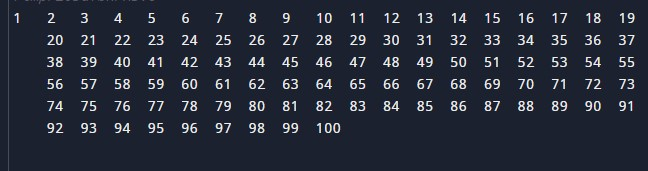In the above program, we have first initialized the required variable.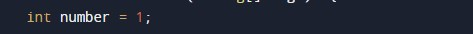• number = it will hold the integer value for the program.

Sending the data to the user-defined function for the generation of series.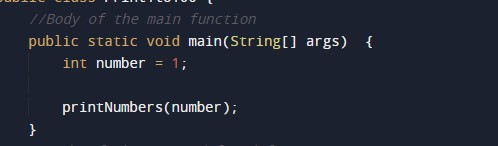Body of the user-defined function to print the series.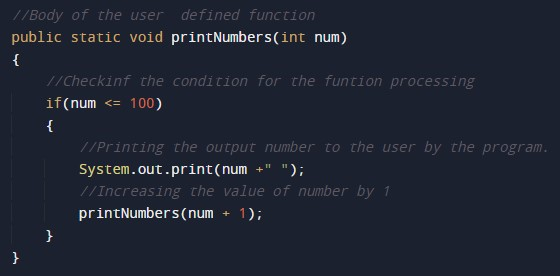Program code to print the list up to the given number 100.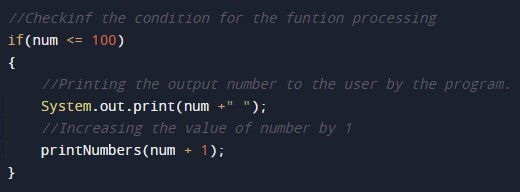In this tutorial we have learn about the Java Program to Print 1 to 100 Without Loop and its application with practical example. I hope you will like this tutorial.